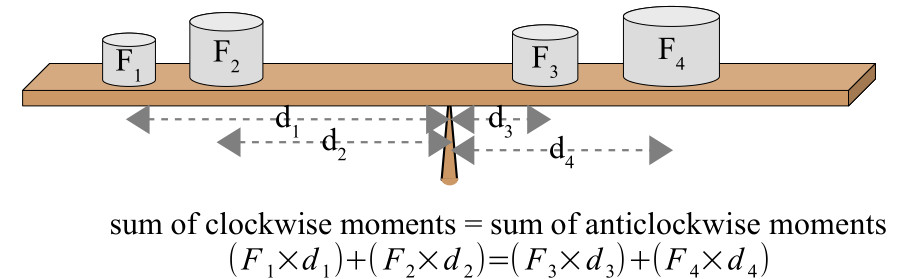Home » Handbook of O Level Physics » UNIT 5: TURNING EFFECT OF FORCES

# UNIT 5: TURNING EFFECT OF FORCES

• Moment of a force: It is the product of force and the perpendicular distance (between the line of action of the force and the pivot).

Moment of a force = F x d

• Moments can be clockwise or anticlockwise.

• The Principle of Moments: For a body in equilibrium, the sum of clockwise moments is equal to the sum of anticlockwise moments.

• Example:• Centre of mass: It is the point through which the whole weight of an object seems to act for any orientation of the object.

• Centre of mass of an object (such as ring) can lie outside the object.

• Experiment to determine centre of mass of a plane lamina

• The marking points are:

• set metal swinging (to ensure minimal friction at pivot)

• allow to come to rest

• use of plumb line from hole

• mark line along plumb line (on metal)

• hang from another hole

• hang from 3 rd hole

• point of intersection of lines is centre of mass

• Stability: It is the ability of an object to regain its original position after it has been tilted slightly.

• Stability can be improved by

• lowering the centre of mass

• increasing the broadness of base